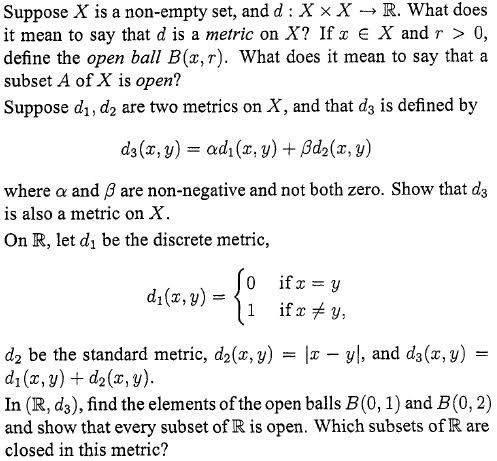# Open/closed subsets of metric space

## Homework Statement## The Attempt at a Solution

I've got through this question up to the last bit.

I've got $B(0,1) = \{0\}$ and $B(0,2) = \{y\in\mathbb{R} : -1<y<1 \}$ (i.e. the open interval (-1,1).)

How do I show that every subset of $\mathbb{R}$ is open ($A \subseteq X$ is open if it contains none if its boundary)

and then find which subsets are closed?

vela
Staff Emeritus
Homework Helper
What definition of the boundary are you using?

What definition of the boundary are you using?

$x\in\partial A$ if for all $r>0$, $B(x,r)$ intersects both $A$ and $A^c$.

Last edited:
vela
Staff Emeritus
Homework Helper
OK, so that means if A is open, for every x in A, you can find some r>0 such that B(x,r) is contained in A.

OK, so that means if A is open, for every x in A, you can find some r>0 such that B(x,r) is contained in A.

OK, so if $A \subseteq \mathbb{R}$ and $x\in A$ then $B(x,1)=\{x\} \subseteq A$ so every subset $A$ of $\mathbb{R}$ is open.

How do I find which subsets are closed?

vela
Staff Emeritus
Homework Helper
The answer should be pretty obvious if you consider the definition of a closed set.

The answer should be pretty obvious if you consider the definition of a closed set.

Is every subset also closed?

If $x\in A^c$ then $B(x,1) = \{x\} \subseteq A^c$

vela
Staff Emeritus